# GRE Subject Test: Math : Polynomials

## Example Questions

1 3 Next →

### Example Question #21 : Polynomials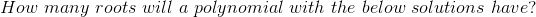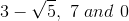Explanation:

If a complex or imaginary root exists, its' complex conjugate must also exist as a root.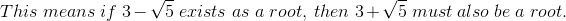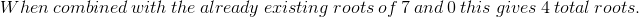### Example Question #21 : Polynomials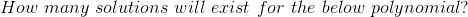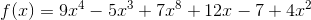Explanation:

Based upon the corollary to the Fundamental Theorem of Algebra, the degree of a function determines the number of solutions/zeros/roots etc. that exist. They may be real, repeated, imaginary or irrational.

In this case, we must first change the function to be in standard form before determining the degree. Standard form means that the largest exponent goes first and the terms are organized by decreasing exponent.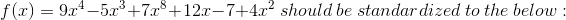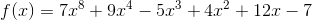Now that the polynomial is in standard form, we see that the degree is 8.

There exists 8 total solutions/roots/zeros for this polynomial.

1 3 Next →

### All GRE Subject Test: Math Resources